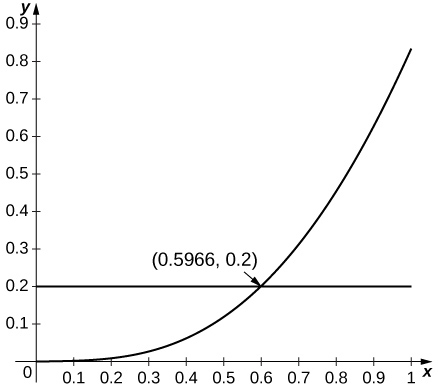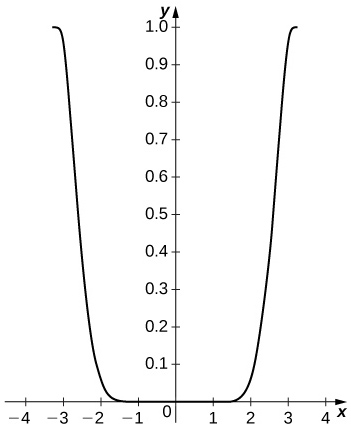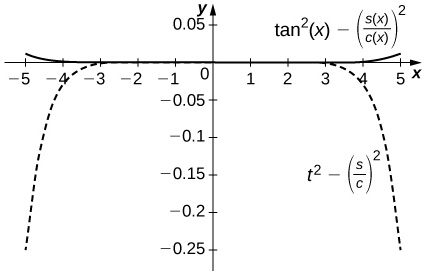$$\newcommand{\id}{\mathrm{id}}$$ $$\newcommand{\Span}{\mathrm{span}}$$ $$\newcommand{\kernel}{\mathrm{null}\,}$$ $$\newcommand{\range}{\mathrm{range}\,}$$ $$\newcommand{\RealPart}{\mathrm{Re}}$$ $$\newcommand{\ImaginaryPart}{\mathrm{Im}}$$ $$\newcommand{\Argument}{\mathrm{Arg}}$$ $$\newcommand{\norm}{\| #1 \|}$$ $$\newcommand{\inner}{\langle #1, #2 \rangle}$$ $$\newcommand{\Span}{\mathrm{span}}$$

# 1.3E: Exercises

$$\newcommand{\vecs}{\overset { \rightharpoonup} {\mathbf{#1}} }$$ $$\newcommand{\vecd}{\overset{-\!-\!\rightharpoonup}{\vphantom{a}\smash {#1}}}$$$$\newcommand{\id}{\mathrm{id}}$$ $$\newcommand{\Span}{\mathrm{span}}$$ $$\newcommand{\kernel}{\mathrm{null}\,}$$ $$\newcommand{\range}{\mathrm{range}\,}$$ $$\newcommand{\RealPart}{\mathrm{Re}}$$ $$\newcommand{\ImaginaryPart}{\mathrm{Im}}$$ $$\newcommand{\Argument}{\mathrm{Arg}}$$ $$\newcommand{\norm}{\| #1 \|}$$ $$\newcommand{\inner}{\langle #1, #2 \rangle}$$ $$\newcommand{\Span}{\mathrm{span}}$$ $$\newcommand{\id}{\mathrm{id}}$$ $$\newcommand{\Span}{\mathrm{span}}$$ $$\newcommand{\kernel}{\mathrm{null}\,}$$ $$\newcommand{\range}{\mathrm{range}\,}$$ $$\newcommand{\RealPart}{\mathrm{Re}}$$ $$\newcommand{\ImaginaryPart}{\mathrm{Im}}$$ $$\newcommand{\Argument}{\mathrm{Arg}}$$ $$\newcommand{\norm}{\| #1 \|}$$ $$\newcommand{\inner}{\langle #1, #2 \rangle}$$ $$\newcommand{\Span}{\mathrm{span}}$$

## Exercise $$\PageIndex{1}$$

In the following exercises, find the Taylor polynomials of degree two approximating the given function centered at the given point.

1. $$\displaystyle f(x)=1+x+x^2$$ at $$\displaystyle a=1$$

2. $$\displaystyle f(x)=1+x+x^2$$ at $$\displaystyle a=−1$$

$$\displaystyle f(−1)=1;f′(−1)=−1;f''(−1)=2;f(x)=1−(x+1)+(x+1)^2$$

3. $$\displaystyle f(x)=cos(2x)$$ at $$\displaystyle a=π$$

4. $$\displaystyle f(x)=sin(2x)$$ at $$\displaystyle a=\frac{π}{2}$$

$$\displaystyle f′(x)=2cos(2x);f''(x)=−4sin(2x);p_2(x)=−2(x−\frac{π}{2})$$

5. $$\displaystyle f(x)=\sqrt{x}$$ at $$\displaystyle a=4$$

6. $$\displaystyle f(x)=lnx$$ at $$\displaystyle a=1$$

$$\displaystyle f′(x)=\frac{1}{x};f''(x)=−\frac{1}{x^2};p_2(x)=0+(x−1)−\frac{1}{2}(x−1)^2$$

7. $$\displaystyle f(x)=\frac{1}{x}$$ at $$\displaystyle a=1$$

8. $$\displaystyle f(x)=e^x$$ at $$\displaystyle a=1$$

$$\displaystyle p_2(x)=e+e(x−1)+\frac{e}{2}(x−1)^2$$

## Exercise $$\PageIndex{2}$$

In the following exercises, verify that the given choice of n in the remainder estimate $$\displaystyle |R_n|≤\frac{M}{(n+1)!}(x−a)^{n+1}$$, where M is the maximum value of $$\displaystyle ∣f^{(n+1)}(z)∣$$ on the interval between a and the indicated point, yields $$\displaystyle |R_n|≤\frac{1}{1000}$$. Find the value of the Taylor polynomial $$\displaystyle p_n$$ of $$\displaystyle f$$ at the indicated point.

1. (\displaystyle \sqrt{10};a=9,n=3\)

2. $$\displaystyle (28)^{1/3};a=27,n=1$$

$$\displaystyle \frac{d^2}{dx^2}x^{1/3}=−\frac{2}{9x^{5/3}}≥−0.00092…$$ when $$\displaystyle x≥28$$ so the remainder estimate applies to the linear approximation $$\displaystyle x^{1/3}≈p_1(27)=3+\frac{x−27}{27}$$, which gives $$\displaystyle (28)^{1/3}≈3+\frac{1}{27}=3.\bar{037}$$, while $$\displaystyle (28)^{1/3}≈3.03658.$$

3. $$\displaystyle sin(6);a=2π,n=5$$

4. $$\displaystyle e^2; a=0,n=9$$

Using the estimate $$\displaystyle \frac{2^{10}}{10!}<0.000283$$ we can use the Taylor expansion of order 9 to estimate $$\displaystyle e^x$$ at $$\displaystyle x=2$$. as $$\displaystyle e^2≈p_9(2)=1+2+\frac{2^2}{2}+\frac{2^3}{6}+⋯+\frac{2^9}{9!}=7.3887$$… whereas $$\displaystyle e^2≈7.3891.$$

5. $$\displaystyle cos(\frac{π}{5});a=0,n=4$$

6. $$\displaystyle ln(2);a=1,n=1000$$

Since $$\displaystyle \frac{d^n}{dx^n}(lnx)=(−1)^{n−1}\frac{(n−1)!}{x^n},R_{1000}≈\frac{1}{1001}$$. One has $$\displaystyle p_{1000}(1)=\sum_{n=1}^{1000}\frac{(−1)^{n−1}}{n}≈0.6936$$ whereas $$\displaystyle ln(2)≈0.6931⋯.$$

## Exercise $$\PageIndex{3}$$

1. Integrate the approximation $$\displaystyle sint≈t−\frac{t^3}{6}+\frac{t^5}{120}−\frac{t^7}{5040}$$ evaluated at $$\displaystyle π$$t to approximate $$\displaystyle ∫^1_0\frac{sinπt}{πt}dt$$.

2. Integrate the approximation $$\displaystyle e^x≈1+x+\frac{x^2}{2}+⋯+\frac{x^6}{720}$$ evaluated at $$\displaystyle −x^2$$ to approximate $$\displaystyle ∫^1_0e^{−x^2}dx.$$

$$\displaystyle ∫^1_0(1−x^2+\frac{x^4}{2}−\frac{x^6}{6}+\frac{x^8}{24}−\frac{x^{10}}{120}+\frac{x^{12}}{720})dx =1−\frac{1^3}{3}+\frac{1^5}{10}−\frac{1^7}{42}+\frac{1^9}{9⋅24}−\frac{1^{11}}{120⋅11}+\frac{1^{13}}{720⋅13}≈0.74683$$ whereas $$\displaystyle ∫^1_0e^{−x^2}dx≈0.74682.$$

## Exercise $$\PageIndex{4}$$

In the following exercises, find the smallest value of n such that the remainder estimate $$\displaystyle |R_n|≤\frac{M}{(n+1)!}(x−a)^{n+1}$$, where M is the maximum value of $$\displaystyle ∣f^{(n+1)}(z)∣$$ on the interval between a and the indicated point, yields $$\displaystyle |R_n|≤\frac{1}{1000}$$ on the indicated interval.

1. $$\displaystyle f(x)=sinx$$ on $$\displaystyle [−π,π],a=0$$

2. $$\displaystyle f(x)=cosx$$ on $$\displaystyle [−\frac{π}{2},\frac{π}{2}],a=0$$

Since $$\displaystyle f^{(n+1)}(z)$$ is $$\displaystyle sinz$$ or $$\displaystyle cosz$$, we have $$\displaystyle M=1$$. Since $$\displaystyle |x−0|≤\frac{π}{2}$$, we seek the smallest n such that $$\displaystyle \frac{π^{n+1}}{2^{n+1}(n+1)!}≤0.001$$. The smallest such value is $$\displaystyle n=7$$. The remainder estimate is $$\displaystyle R_7≤0.00092.$$

3. $$\displaystyle f(x)=e^{−2x}$$ on $$\displaystyle [−1,1],a=0$$

4. $$\displaystyle f(x)=e^{−x}$$ on $$\displaystyle [−3,3],a=0$$

Since $$\displaystyle f^{(n+1)}(z)=±e^{−z}$$ one has $$\displaystyle M=e^3$$. Since $$\displaystyle |x−0|≤3$$, one seeks the smallest n such that $$\displaystyle \frac{3^{n+1}e^3}{(n+1)!}≤0.001$$. The smallest such value is $$\displaystyle n=14$$. The remainder estimate is $$\displaystyle R_{14}≤0.000220.$$

## Exercise $$\PageIndex{5}$$

In the following exercises, the maximum of the right-hand side of the remainder estimate $$\displaystyle |R_1|≤\frac{max|f''(z)|}{2}R^2$$ on $$\displaystyle [a−R,a+R]$$ occurs at a or $$\displaystyle a±R$$. Estimate the maximum value of R such that $$\displaystyle \frac{max|f''(z)|}{2}R^2≤0.1$$ on $$\displaystyle [a−R,a+R]$$ by plotting this maximum as a function of I.

1. $$\displaystyle e^x$$ approximated by $$\displaystyle 1+x,a=0$$

2. $$\displaystyle sinx$$ approximated by $$\displaystyle x, a=0$$

Since $$\displaystyle sinx$$ is increasing for small $$\displaystyle x$$ and since $$\displaystyle sin''x=−sinx$$, the estimate applies whenever $$\displaystyle R^2sin(R)≤0.2$$, which applies up to $$\displaystyle R=0.596.$$3. $$\displaystyle lnx$$ approximated by $$\displaystyle x−1,a=1$$

4. $$\displaystyle cosx$$ approximated by $$\displaystyle 1,a=0$$

Since the second derivative of $$\displaystyle cosx$$ is $$\displaystyle −cosx$$ and since $$\displaystyle cosx$$ is decreasing away from $$\displaystyle x=0$$, the estimate applies when $$\displaystyle R^2cosR≤0.2$$ or $$\displaystyle R≤0.447$$.## Exercise $$\PageIndex{6}$$

In the following exercises, find the Taylor series of the given function centered at the indicated point.

1. $$\displaystyle x^4$$ at $$\displaystyle a=−1$$

2. $$\displaystyle 1+x+x^2+x^3$$ at $$\displaystyle a=−1$$

$$\displaystyle (x+1)^3−2(x+1)^2+2(x+1)$$

3. $$\displaystyle sinx$$ at $$\displaystyle a=π$$

4. $$\displaystyle cosx$$ at $$\displaystyle a=2π$$

Values of derivatives are the same as for $$\displaystyle x=0$$ so $$\displaystyle cosx=\sum_{n=0}^∞(−1)^n\frac{(x−2π)^{2n}}{(2n)!}$$

5. $$\displaystyle sinx$$ at $$\displaystyle x=\frac{π}{2}$$

6. $$\displaystyle cosx$$ at $$\displaystyle x=\frac{π}{2}$$

$$\displaystyle cos(\frac{π}{2})=0,−sin(\frac{π}{2})=−1$$ so $$\displaystyle cosx=\sum_{n=0}^∞(−1)^{n+1}\frac{(x−\frac{π}{2})^{2n+1}}{(2n+1)!}$$, which is also $$\displaystyle −cos(x−\frac{π}{2})$$.

7. $$\displaystyle e^x$$ at $$\displaystyle a=−1$$

8. $$\displaystyle e^x$$ at $$\displaystyle a=1$$

The derivatives are $$\displaystyle f^{(n)}(1)=e$$ so $$\displaystyle e^x=e\sum_{n=0}^∞\frac{(x−1)^n}{n!}.$$

9. $$\displaystyle \frac{1}{(x−1)^2}$$ at $$\displaystyle a=0$$ (Hint: Differentiate $$\displaystyle \frac{1}{1−x}$$.)

10. $$\displaystyle \frac{1}{(x−1)^3}$$ at $$\displaystyle a=0$$

$$\displaystyle \frac{1}{(x−1)^3}=−(\frac{1}{2})\frac{d^2}{dx^2}\frac{1}{1−x}=−\sum_{n=0}^∞(\frac{(n+2)(n+1)x^n}{2})$$

11. $$\displaystyle F(x)=∫^x_0cos(\sqrt{t})dt;f(t)=\sum_{n=0}^∞(−1)^n\frac{t^n}{(2n)!}$$ at a=0 (Note: $$\displaystyle f$$ is the Taylor series of $$\displaystyle cos(\sqrt{t}).)$$

## Exercise $$\PageIndex{7}$$

In the following exercises, compute the Taylor series of each function around $$\displaystyle x=1$$.

1. $$\displaystyle f(x)=2−x$$

$$\displaystyle 2−x=1−(x−1)$$

2. $$\displaystyle f(x)=x^3$$

3. $$\displaystyle f(x)=(x−2)^2$$

$$\displaystyle ((x−1)−1)^2=(x−1)^2−2(x−1)+1$$

4. $$\displaystyle f(x)=lnx$$

5. $$\displaystyle f(x)=\frac{1}{x}$$

$$\displaystyle \frac{1}{1−(1−x)}=\sum_{n=0}^∞(−1)^n(x−1)^n$$

6. $$\displaystyle f(x)=\frac{1}{2x−x^2}$$

7. $$\displaystyle f(x)=\frac{x}{4x−2x^2−1}$$

$$\displaystyle x\sum_{n=0}^∞2^n(1−x)^{2n}=\sum_{n=0}^∞2^n(x−1)^{2n+1}+\sum_{n=0}^∞2^n(x−1)^{2n}$$

8. $$\displaystyle f(x)=e^{−x}$$

9. $$\displaystyle f(x)=e^{2x}$$

$$\displaystyle e^{2x}=e^{2(x−1)+2}=e^2\sum_{n=0}^∞\frac{2^n(x−1)^n}{n!}$$

## Exercise $$\PageIndex{8}$$

In the following exercises, identify the value of x such that the given series $$\displaystyle \sum_{n=0}^∞a_n$$ is the value of the Maclaurin series of $$\displaystyle f(x)$$ at $$\displaystyle x$$. Approximate the value of $$\displaystyle f(x)$$ using $$\displaystyle S_{10}=\sum_{n=0}^{10}a_n$$.

1. $$\displaystyle \sum_{n=0}^∞\frac{1}{n!}$$

2. $$\displaystyle sum_{n=0}^∞\frac{2^n}{n!}$$

$$\displaystyle x=e^2;S_{10}=\frac{34,913}{4725}≈7.3889947$$

3. $$\displaystyle \sum_{n=0}^∞\frac{(−1)^n(2π)^{2n}}{(2n)!}$$

4. $$\displaystyle \sum_{n=0}^∞\frac{(−1)^n(2π)^{2n+1}}{(2n+1)!}$$

$$\displaystyle sin(2π)=0;S_{10}=8.27×10^{−5}$$

## Exercise $$\PageIndex{9}$$

The following exercises make use of the functions $$\displaystyle S_5(x)=x−\frac{x^3}{6}+\frac{x^5}{120}$$ and $$\displaystyle C_4(x)=1−\frac{x^2}{2}+\frac{x^4}{24}$$ on $$\displaystyle [−π,π]$$.

1. Plot $$\displaystyle sin^2x−(S_5(x))^2$$ on $$\displaystyle [−π,π]$$. Compare the maximum difference with the square of the Taylor remainder estimate for $$\displaystyle sinx.$$

2. Plot $$\displaystyle cos^2x−(C_4(x))^2$$ on $$\displaystyle [−π,π]$$. Compare the maximum difference with the square of the Taylor remainder estimate for $$\displaystyle cosx$$.

The difference is small on the interior of the interval but approaches $$\displaystyle 1$$ near the endpoints. The remainder estimate is $$\displaystyle |R_4|=\frac{π^5}{120}≈2.552.$$3. Plot $$\displaystyle |2S_5(x)C_4(x)−sin(2x)|$$ on $$\displaystyle [−π,π]$$.

4. Compare $$\displaystyle \frac{S_5(x)}{C_4(x)}$$ on $$\displaystyle [−1,1]$$ to $$\displaystyle tanx$$. Compare this with the Taylor remainder estimate for the approximation of $$\displaystyle tanx$$ by $$\displaystyle x+\frac{x^3}{3}+\frac{2x^5}{15}$$.

The difference is on the order of $$\displaystyle 10^{−4}$$ on $$\displaystyle [−1,1]$$ while the Taylor approximation error is around $$\displaystyle 0.1$$ near $$\displaystyle ±1$$. The top curve is a plot of $$\displaystyle tan^2x−(\frac{S_5(x)}{C_4(x)})^2$$ and the lower dashed plot shows $$\displaystyle t^2−(\frac{S_5}{C_4})^2$$.5. Plot $$\displaystyle e^x−e_4(x)$$ where $$\displaystyle e_4(x)=1+x+\frac{x^2}{2}+\frac{x^3}{6}+\frac{x^4}{24}$$ on $$\displaystyle [0,2]$$. Compare the maximum error with the Taylor remainder estimate.

## Exercise $$\PageIndex{10}$$

1. (Taylor approximations and root finding.) Recall that Newton’s method $$\displaystyle x_{n+1}=x_n−\frac{f(x_n)}{f'(x_n)}$$ approximates solutions of $$\displaystyle f(x)=0$$ near the input $$\displaystyle x_0$$.

a. If $$\displaystyle f$$ and $$\displaystyle g$$ are inverse functions, explain why a solution of $$\displaystyle g(x)=a$$ is the value $$\displaystyle f(a)$$ of $$\displaystyle f$$.

b. Let $$\displaystyle p_N(x)$$ be the $$\displaystyle Nth$$ degree Maclaurin polynomial of $$\displaystyle e^x$$. Use Newton’s method to approximate solutions of $$\displaystyle p_N(x)−2=0$$ for $$\displaystyle N=4,5,6.$$

c. Explain why the approximate roots of $$\displaystyle p_N(x)−2=0$$ are approximate values of $$\displaystyle ln(2).$$

b. The following are the $$\displaystyle x_n$$ values after $$\displaystyle 10$$ iterations of Newton’s method to approximation a root of $$\displaystyle p_N(x)−2=0$$: for $$\displaystyle N=4,x=0.6939...;$$ for $$\displaystyle N=5,x=0.6932...;$$ for $$\displaystyle N=6,x=0.69315...;.$$ (Note: $$\displaystyle ln(2)=0.69314...$$)

## Exercise $$\PageIndex{11}$$

In the following exercises, use the fact that if $$\displaystyle q(x)=\sum_{n=1}^∞a_n(x−c)^n$$ converges in an interval containing $$\displaystyle c$$, then $$\displaystyle \lim_{x→c}q(x)=a_0$$ to evaluate each limit using Taylor series.

1. $$\displaystyle \lim_{x→0}\frac{cosx−1}{x^2}$$

2. $$\displaystyle \lim_{x→0}\frac{ln(1−x^2)}{x^2}$$

$$\displaystyle \frac{ln(1−x^2)}{x^2}→−1$$
3. $$\displaystyle \lim_{x→0}\frac{e^{x^2}−x^2−1}{x^4}$$
4. $$\displaystyle \lim_{x→0^+}\frac{cos(\sqrt{x})−1}{2x}$$
$$\displaystyle \frac{cos(\sqrt{x})−1}{2x}≈\frac{(1−\frac{x}{2}+\frac{x^2}{4!}−⋯)−1}{2x}→−\frac{1}{4}$$